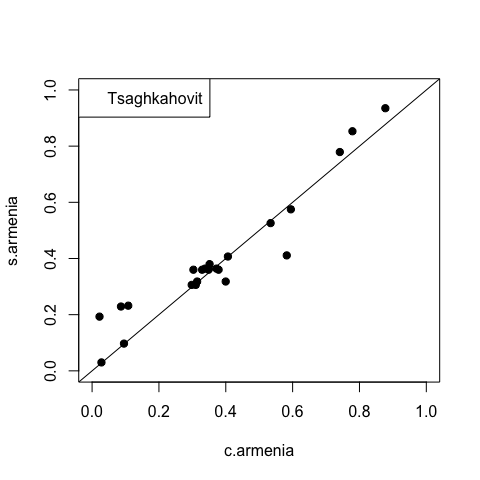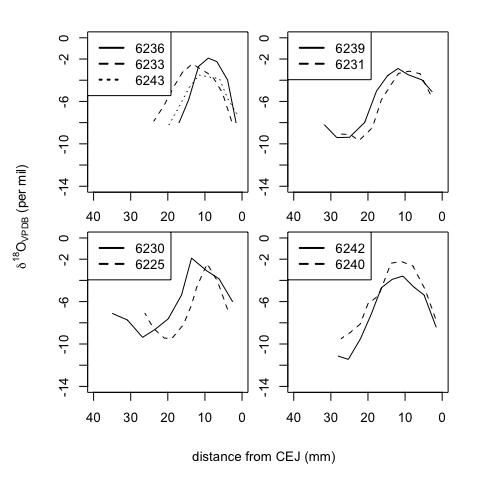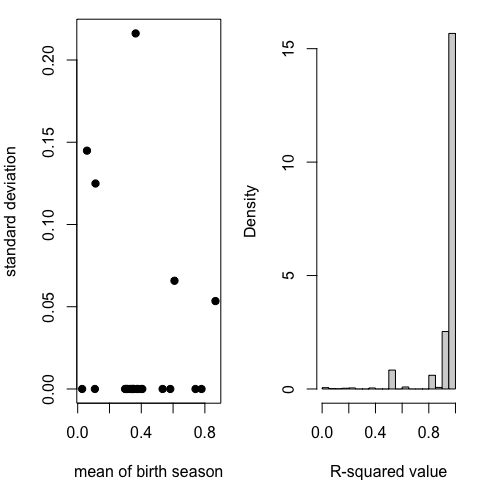# SCEM_example

## Install the package

#Install the github package
#devtools::install_github("kserkcho/SCEM")
library(SCEM)
#>
#> Attaching package: 'SCEM'
#> The following object is masked from 'package:stats':
#>
#>     kernel

data(armenia)
oxy <- armenia
armenia = split(oxy,f = oxy$ID) ## Implement the SCEM and Cosine method on the Armenia data results = SCEM(armenia,bandwidth = -0.33) cosine = makeFits(armenia,method ="OLS") rownames(cosine) = 1:nrow(cosine) results$results$Cosine = cosine$birth
dif = abs(results$results$Cosine - results$results$Season)
results$results$Difference = apply(cbind(dif,(1-dif)),1,min)
results$results$R = cosine$Pearson rrr.a = results$results[order(results$results$Cluster),]

# Storing the birth seasonality estimates from two methods
c.armenia = results$results$Cosine
s.armenia = results$results$Season

## Prepare relevant plots for the above analysis


# Plotting the estimates of birth seasonality from the two methods
plot(c.armenia,s.armenia,xlim = c(0,1),ylim = c(0,1),pch = 19)
abline(0,1)
legend("topleft","Tsaghkahovit")
title(xlab = "estimated birth seasonality from cosine method",ylab = "estimated birth seasonality from SCEM",outer = TRUE, line = 2)# Plotting the graphs of the clusters for Armenia data

gnum = max(results$results$Cluster)
oldpar = par(mar=c(1.5,1.5,1,1), mfrow=c(2,2),oma = c(4, 4, 1, 1))
for(i in 1:gnum){
if(length(results$groups[[i]])>1){ cc = as.character() plot(c(0,40),c(-14,0),type = "n",axes = T,xlab = "",ylab = "",xlim = rev(range(c(0,40)))) for(j in 1:length(results$groups[[i]])){
tt = armenia[[results$groups[[i]][j]]] lines(tt$distance,tt$oxygen,lty = j) cc = c(cc,paste(tt$lab,tt$ID,sep = "")) } legend("topleft",legend = cc,lty = c(1:j),lwd = 2) } } title(xlab = "distance from CEJ (mm)",ylab = expression(delta^18*O[VPDB]*' (per mil)'),outer = TRUE, line = 2)par(oldpar) ## Use Armenia data to show the sensitivity to initialization (for Cosine method) # Fix the initial values of amplitude and intercept amp = seq(1,10,by=0.5) int = seq(-25,0,by=0.5) # Find the estimate of birth seasonality, period, delay and the R^2 for all cases cosfit.birth = array(0,dim = c(length(amp),length(int),length(armenia))) cosfit.period = array(0,dim = c(length(amp),length(int),length(armenia))) cosfit.delay = array(0,dim = c(length(amp),length(int),length(armenia))) cosfit.R = array(0,dim = c(length(amp),length(int),length(armenia))) for (i in 1:length(amp)){ for (j in 1:length(int)){ dd = makeFits(armenia,amp[i],int[j],method="initial") cosfit.birth[i,j,] = dd$birth
cosfit.period[i,j,] = dd$X cosfit.delay[i,j,] = dd$x0
cosfit.R[i,j,] = dd\$Pearson
}
}
birth.means = numeric(length(armenia))
birth.var = numeric(length(armenia))
for (i in 1:length(armenia)){
birth.means[i] = mean(c(cosfit.birth[,,i]))
birth.var[i] = var(c(cosfit.birth[,,i]))
}

# Plots of the model fits to show the sensitivity to initial conditions

oldpar2= par(mfrow = c(1,2),mar = c(4,4,1,1))
plot(birth.means,sqrt(birth.var),xlab = "mean of birth season",ylab = "standard deviation",pch = 19)
hist(c(cosfit.R^2),25,freq = F,xlab = "R-squared value",main = "")par(oldpar2)